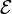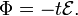# Gauss (unit)

In physics, gauss (symbol G) is the unit of strength of magnetic flux density |B| (also known as magnetic induction). The gauss belongs to the Gaussian and emu (electromagnetic) systems of units, which are cgs (centimeter-gram-second) systems. The unit is related to the SI unit tesla (T) as follows

1 G ≡ 1 Mx/cm2 = 10−4  T,

here Mx (maxwell) is the Gaussian unit for magnetic flux.

The unit is named in honor of the German mathematician and physicist Carl Friedrich Gauss.

## Definition

The gauss is defined through an electromotive force$\mathcal{E}$ induced by a change in magnetic field B. For constant surface S and uniform rate of decrease of |B|, Faraday's law takes the simple form$|\mathbf{B}| = \frac{\Phi}{S} = - \frac{t\,\mathcal{E}}{S} ,$

where Φ is the magnetic flux passing through S and uniform rate of decrease means linearity in time:$\Phi = - t \mathcal{E}.$ Hence, gauss is equal to maxwell per unit surface, where maxwell (symbol Mx) is the Gaussian unit for Φ, and |B| is a flux density.

In Gaussian units S is in cm2, time t in s,$\mathcal{E}$ in abV ( = 10−8 volt), |B| in G, and Φ in Mx:

1 G = 1 Mx/cm2 = 1 abV•s/cm2

## Related unit

• The oersted is the Gaussian unit of strength of a magnetic field |H|. The oersted is defined by means of an electric current giving the field H.